Courses

# NCERT Solutions - Solid State (Part - 1) Class 12 Notes | EduRev

## Class 12 : NCERT Solutions - Solid State (Part - 1) Class 12 Notes | EduRev

The document NCERT Solutions - Solid State (Part - 1) Class 12 Notes | EduRev is a part of the Class 12 Course Chemistry Class 12.
All you need of Class 12 at this link: Class 12

Intext Questions

Q.1.1. Why are Solids rigid?
Ans: The intermolecular forces of attraction that are present in solids are very strong. The constituent particles of solids cannot move from their positions i.e., they have fixed positions. However, they can oscillate about their mean positions. This is the reason that solids are rigid.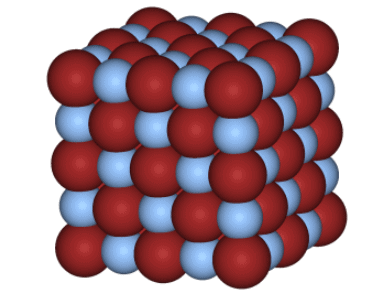Structure of SolidsQ.1.2. Why do Solids have a definite volume?
Ans: The inter-molecular forces of attraction that are present in solids are very strong. The constituent particles of solids have fixed positions i.e., they are rigid. Hence, solids have a definite volume.

Q.1.3. Classify the following as Amorphous or Crystalline Solids:

Polyurethane, Naphthalene, Benzoic acid, Teflon, Potassium Nitrate, Cellophane, Polyvinyl Chloride, Fibreglass, Copper.
Ans:

• Amorphous solids:
Polyurethane, Teflon, cellophane, polyvinyl chloride, fibre glass
• Crystalline solids:
Naphthalene, benzoic acid, potassium nitrate, copper

Q.1.4. Why is glass considered a supercooled liquid?

Ans: Similar to liquids, glass has a tendency to flow, though very slowly. Therefore, glass is considered as a supercooled liquid. This is the reason that glass windows and doors are slightly thicker at the bottom than at the top.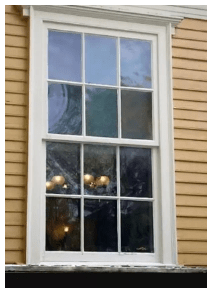Glass Window

Q.1.5. Refractive index of a solid is observed to have the same value along all directions. Comment on the nature of this solid. Would it show cleavage property?

Ans: An isotropic solid has the same value of physical properties when measured along different directions. Therefore, the given solid, having the same value of refractive index along all directions, is isotropic in nature. Hence, the solid is an amorphous solid.

When an amorphous solid is cut with a sharp-edged tool, it cuts into two pieces with irregular surfaces.

Q.1.6. Classify the following solids in different categories based on the nature of intermolecular forces operating in them:
Potassium sulphate, tin, benzene, urea, ammonia, water, zinc sulphide, graphite, rubidium, argon, silicon carbide.
Ans:

• Potassium sulphate → Ionic solid
• Tin → Metallic solid
• Benzene → Molecular (non-polar) solid
• Urea → Polar molecular solid
• Ammonia → Polar molecular solid
• Water → Hydrogen bonded molecular solid
• Zinc sulphide → Ionic solid
• Graphite → Covalent or network solid
• Rubidium → Metallic solid
• Argon → Non-polar molecular solid
• Silicon carbide → Covalent or network solid

Q.1.7. Solid A is a very hard electrical insulator in solid as well as in molten state and melts at extremely high temperature. What type of solid is it?
Ans: The given properties are the properties of a covalent or network solid. Therefore, the given solid is a covalent or network solid. Examples of such solids include diamond (C) and quartz (SiO2).

Q.1.8. Ionic solids conduct electricity in molten state but not in solid state. Explain.
Ans: In ionic compounds, electricity is conducted by ions. In solid state, ions are held together by strong electrostatic forces and are not free to move about within the solid. Hence, ionic solids do not conduct electricity in solid state. However, in molten state or in solution form, the ions are free to move and can conduct electricity.

Q.1.9. What type of solids are electrical conductors, malleable and ductile?
Ans: Metallic solids are electrical conductors, malleable, and ductile.

Q.1.10. Give the significance of a ‘lattice point’.
Ans: The significance of a lattice point is that each lattice point represents one constituent particle of a solid which may be an atom, a molecule (a group of atoms), or an ion.

Q.1.11. Name the parameters that characterize a unit cell.
Ans:
Following parameters characterized  the unit cell:
(a) Its dimensions along the three edges a, b, and c.
These edges may or may not be equal.
(b) Angles between the edges
These are the angle ∝ (between edges b and c), β (between edges a and c), and γ (between edges a and b).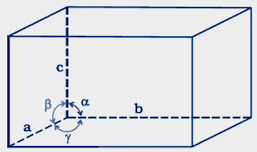Parameters of a Unit Cell

Q.1.12. Distinguish between
(i) Hexagonal and Monoclinic unit cells
(ii) Face-centred and End-centred unit cells.
Ans:
(i) Hexagonal and Monoclinic unit cells

• Hexagonal unit cell:
For a hexagonal unit cell,
a = b ≠ c, and α = β = c, and α = β = 90°
γ = 120°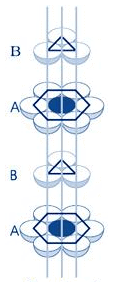Hexagonal Packing

• Monoclinic unit cell:
For a monoclinic cell,
a ≠ b ≠ c, and α = γ = 90°
β ≠ 90°

(ii) Face-centred and End-centred unit cells

• Face-centred unit cells:
In a face-centred unit cell, the constituent particles are present at the corners and one at the centre of each face.
• End-centred unit cells:
An end-centred unit cell contains particles at the corners and one at the centre of any two opposite faces.

Q.1.13. Explain how much portion of an atom located at the corner and body-centre of a cubic unit cell is part of its neighbouring unit cell.

Ans:
(i) An atom located at the corner of a cubic unit cell is shared by eight adjacent unit cells.
Therefore,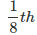portion of the atom is shared by one unit cell.

(ii) An atom located at the body centre of a cubic unit cell is not shared by its neighbouring unit cell. Therefore, the atom belongs only to the unit cell in which it is present i.e., its contribution to the unit cell is 1.

Q.1.14. What is the two dimensional coordination number of a molecule in the square close-packed layer?
Ans:-  In square close-packed layer, a molecule is in contact with four of its neighbours. Therefore, the two-dimensional coordination number of a molecule in square close-packed layer is 4.

Q.1.15. A compound forms hexagonal close-packed structure. What is the total number of voids in 0.5 mol of it? How many of these are tetrahedral voids?
Ans:
Number of close-packed particles = 0.5 × 6.022 × 1023 = 3.011 × 1023
Therefore, the number of octahedral voids = 3.011 × 1023
And, number of tetrahedral voids = 2 × 3.011 × 1023 = 6.022 ×1023
Therefore, the total number of voids = 3.011 × 1023 6.022 × 1023 = 9.033 × 1023

Q. 1.16. A compound is formed by two elements M and N. The element N forms ccp and atoms of M occupy 1/3rd of tetrahedral voids. What is the formula of the compound?
Ans: The ccp lattice is formed by the atoms of the element N.

Here, the number of tetrahedral voids generated is equal to twice the number of atoms of the element N.

According to the question, the atoms of element M occupy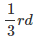of the tetrahedral voids. Therefore, the number of atoms of M is equal to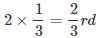of the number of atoms of N.
Therefore, the ratio of the number of atoms of M to that of N is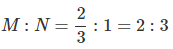Thus, the formula of the compound is M2N3.

Q.1.17. Which of the following lattices has the highest packing efficiency:
(a) Simple cubic.
(b) Body-centred cubic.
(c) Hexagonal close-packed lattice.
Ans: Hexagonal close-packed lattice has the highest packing efficiency of 74%. The packing efficiencies of simple cubic and body-centred cubic lattices are 52.4% and 68% respectively.

Q.1.18. An element with molar mass 2.7 × 10-2 kg mol-1 forms a cubic unit cell with edge length 405 pm. If its density is 2.7 × 103 kg m−3, what is the nature of the cubic unit cell?
Ans:  It is given that density of the element, d = 2.7 × 103 kg m−3

Molar mass, M = 2.7 × 10−2 kg mol−1
Edge length, a = 405 pm = 405 × 10−12 m
= 4.05 × 10−10 m
It is known that, Avogadro’s number, NA = 6.022 × 1023 mol−1

Applying the relation,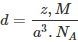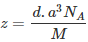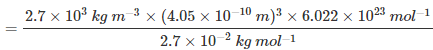=4.004
=4

This implies that four atoms of the element are present per unit cell. Hence, the unit cell is face-centred cubic (fcc) or cubic close-packed (ccp).

Q.1.19. What type of defect can arise when a solid is heated? Which physical property is affected by it and in what way?

Ans:-  When a solid is heated, vacancy defect can arise.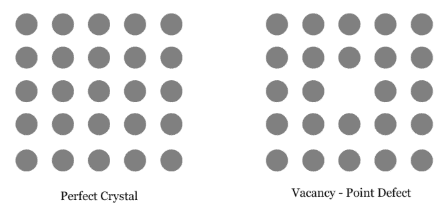A solid crystal is said to have vacancy defect when some of the lattice sites are vacant. Vacancy defect leads to a decrease in the density of the solid.

Q.1.20. What type of stoichiometric defect is shown by:
(i) ZnS
(ii) AgBr
Ans:
(i) ZnS shows Frenkel defect.
(ii) AgBr shows Frenkel defect as well as Schottky defect.

Q.1.21. Explain how vacancies are introduced in an ionic solid when a cation of higher valence is added as an impurity in it.
Ans: When a cation of higher valence is added to an ionic solid as an impurity to it, the cation of higher valence replaces more than one cation of lower valence so as to keep the crystal electrically neutral. As a result, some sites become vacant.

Example: When Sr2+  is added to NaCl, each Sr2+ ion replaces two Na ions. However, one Sr2+ ion occupies the site of one Na+  ion and the other site remains vacant. Hence, vacancies are introduced.

Q.1.22. Ionic solids, which have anionic vacancies due to metal excess defect, develop colour. Explain with the help of a suitable example.
Ans: The colour develops because of the presence of electrons in the anionic sites. These electrons absorb energy from the visible part of radiation and get excited.

Example: When crystals of NaCl are heated in an atmosphere of sodium vapours, the sodium atoms get deposited on the surface of the crystal and the chloride ions from the crystal diffuse to the surface to form NaCl with the deposited Na atoms. During this process, the Na atoms on the surface lose electrons to form Na+  ions and the released electrons diffuse into the crystal to occupy the vacant anionic sites. These electrons get excited by absorbing energy from the visible light and impart yellow colour to the crystals.

Q.1.23. A group 14 element is to be converted into n-type semiconductor by doping it with a suitable impurity. To which group should this impurity belong?
Ans: An n-type semiconductor conducts because of the presence of extra electrons. Therefore, a group 14 element can be converted to n-type semiconductor by doping it with a group 15 element.

Q.1.24. What type of substances would make better permanent magnets, ferromagnetic or ferrimagnetic. Justify your answer.
Ans: Ferromagnetic substances would make better permanent magnets.

• In a solid-state, the metal ions of ferromagnetic substances are grouped together into small regions. These regions are called domains and each domain acts as a tiny magnet. In an unmagnetized piece of a ferromagnetic substance, the domains are randomly oriented. As a result, the magnetic moments of the domains get canceled.
• However, when the substance is placed in a magnetic field, all the domains get oriented in the direction of the magnetic field and a strong magnetic effect is produced. The ordering of the domains persists even after the removal of the magnetic field. Thus, the ferromagnetic substance becomes a permanent magnet.
Exercise Questions

Q.1.1. Define the term 'Amorphous'. Give a few examples of amorphous solids.
Ans: Amorphous solids are the solids whose constituent particles are of irregular shapes and have short range order. These solids are isotropic in nature and melt over a range of temperature. Therefore, amorphous solids are sometimes called pseudo solids or supercooled liquids. They do not have definite heat of fusion. When cutting with a sharp-edged tool, they cut into two pieces with irregular surfaces.
Examples: glass, rubber, and plastic.

Q.1.2. What makes a glass different from a solid such as quartz? Under what conditions could quartz be converted into glass?
Ans: The arrangement of the constituent particles makes glass different from quartz. In glass, the constituent particles have short-range order, but in quartz, the constituent particles have both long-range and short-range orders.
Quartz can be converted into glass by heating and then cooling it rapidly.

Offer running on EduRev: Apply code STAYHOME200 to get INR 200 off on our premium plan EduRev Infinity!

## Chemistry Class 12

121 videos|250 docs|199 tests

,

,

,

,

,

,

,

,

,

,

,

,

,

,

,

,

,

,

,

,

,

;